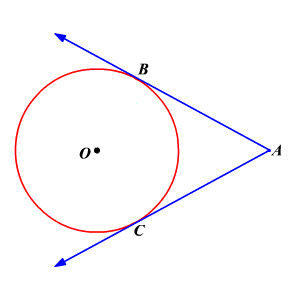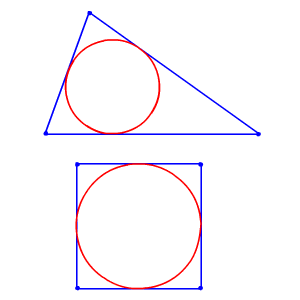# Congruent Tangents and Circumscribed Polygons

## Congruent Tangents

A tangent to a circle is a straight line which touches the circle at only one point. This point is called the point of tangency.

More than one line can be tangent to the same circle. If two segments from the same exterior point are tangent to a circle, then they are congruent.In the figure, $\stackrel{¯}{AB}$ and $\stackrel{¯}{AC}$ are tangents to the circle $O$ . Since $\stackrel{¯}{AB}$ and $\stackrel{¯}{AC}$ are drawn from the same exterior point $A$ and are tangent to circle $O$ , $\stackrel{¯}{AB}$ is congruent to $\stackrel{¯}{AC}$ .

## Circumscribed Polygons

Polygons can be circumscribed about a circle, or the circle is inscribed in the polygon.When a polygon is circumscribed in a circle, the vertices of the polygon do not lie on the circle and its sides are tangent to the circle. The circumscribed polygon intersects at the midpoint of each side to the inscribed circle.